Inductive Solutions, Inc.
380 Rector Place, Suite 4A, New York, New York 10280

Email  Telephone: +1 (212)945.0630

Home

Products and Services
Software Products
Recommended Books

Bibliography and White Papers

## RunRandom: Quasi Monte Carlo in Excel and VBA

RunRandom VBA and Excel spreadsheet add-in functions generate multi-dimensional quasi random vectors.  Components of these vectors -- called quasi random numbers -- can be used in various Monte Carlo applications, such as:

• Evaluation of multi-dimensional integrals.
• Financial evaluation of securities.
• Multivariate stochastic optimization.
• Stochastic differential equations.
• System simulation.
In many Monte Carlo and simulation applications, using quasi random numbers -- instead of the usual pseudo random numbers provided by C, VBA, Excel, and other programming languages -- provide -- on the average -- faster convergence. This means that you can get the same result in less time with less computation. Conversely, for a set simulation time, using quasi-random vectors provide -- on the average -- more accurate answers for Monte Carlo applications. See, for example:
• Hoogland, Jiri, Fred James, and Ronald Kleiss. Quasi-Monte Carlo, discrepancies and error estimates. Monte Carlo and Quasi-Monte Carlo Methods 1996. Springer New York, 1998. 266-276.
• Ramamoorthy, Subramanian, et al. "Low-discrepancy curves and efficient coverage of space." Algorithmic Foundation of Robotics VII. Springer Berlin Heidelberg, 2008. 203-218.

RunRandom is based on a very fast pre-computed version of the Richtmeyer scheme. It requires Microsoft Excel 2010 and later (64-bit installations preferred). RunRandom generates quasi-random vectors up to 5,000 dimensions. The RunRandom Student Version is limited to 100 dimensions. Purchase Student Version

Integration with Excel and VBA. RunRandom Excel formulas follow the same conventions as the Excel =RAND() function and the VBA RND function. The difference is that instead of an empty argument, the RunRandom function calls specify a dimension. For example, suppose we want to specify a random vector in one row and two columns. Using the standard Excel rand() function, enter formulas in cells A1 and B1:
 A B 1 =RAND() =RAND()

For RunRandom, we use an array formula on the spreadsheet range A1:B1 (use Control-Shift-Enter to enter the array formula):
 A B 1 {=RRAND(1, 2)} {=RRAND(1, 2)}

The array formula {=RRAND(1, 2)} specifies a 2-dimensional quasi-random vector of one row and two columns. Similarly, the array formula {=rrand(2,3)} specifies a 6-dimensional quasi-random vector of that can be used to fill a region of 2 rows and 3 columns.

Note that all RunRandom functions are callable from other VBA modules as well.

The Monte Carlo and and Computer-Generated "Random" Numbers   How can one assess the future?  One way is to simulate a large number of alternative future scenarios by computer and seeing what the most likely scenario is by averaging all future scenarios together.  This is the idea behind Monte Carlo Simulation methods.

Computer generated numbers are not really random but only appear so when subjected to certain statistical tests.  Quasi random numbers (components of quasi random vectors) are based on properties of prime numbers and prime polynomial fields. They are also called low discrepancy sequences because when they are generated, they fill in multi-dimensional rectangular regions evenly and uniformly.  Pseudo random numbers -- those provided by built-in functions in C, VBA, Excel, and other programming languages -- do not have this multi-dimensional "low discrepancy" property. In multiple dimensions, sets of pseudo random numbers leave gaps and holes in multiple dimensional space.

Examples of Quasi Monte Carlo Quasi Monte Carlo methods use samples of quasi random vectors instead of samples of sets of pseudo random numbers. For example:

• To evaluate an N-dimensional multi-dimensional integral:
1. Generate an N-dimensional quasi random vector.
2. Use the vector components as arguments to an N-dimensional multivariate integrand function.
3. Evaluate the N-dimensional multivariate integrand function.
4. Repeat with another N-dimensional quasi random vector.
5. Compute the average -- over integrand function evaluations -- for the final result.
• To evaluate a financial security over a time period T:
1. Decompose the time period T into N increments of Δt duration.
2. Generate an N-dimensional quasi random vector.
3. Use the vector components to generate an interest rate or market "scenario" for each Δt.
4. Repeat with another N-dimensional quasi random vector.
5. Compute the Average -- over all scenarios -- for the final result.

For example, suppose we want to compute the 2-dimensional integral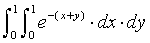via Monte Carlo integration. (This integral is evaluated via calculus as exp(-2)-2*exp(-1)+1 = 0.399576401... . When comparing the performance of 100 quasi random vectors (x,y) with 100 pseudo random pairs, one simulation run showed that the relative error for quasi Monte Carlo was about 42.5% of the pseudo Monte Carlo error (3.06% vs. 7.20%); for 500 random vectors (x,y), the relative error for quasi Monte Carlo error was about 6.5% of the pseudo Monte Carlo error (0.12% vs. 1.84%). The details are summarized in this spreadsheet; the spreadsheet formulas are shown here ).

Comparison of Quasi Random Vectors with Sets of Pseudo Random Numbers Here is an example that compares a set of 100 two dimensional quasi random vectors generated with {=rrand(1,2)} (left chart) with a set of 100 pairs of pseudo random numbers generated with =rand() (right chart):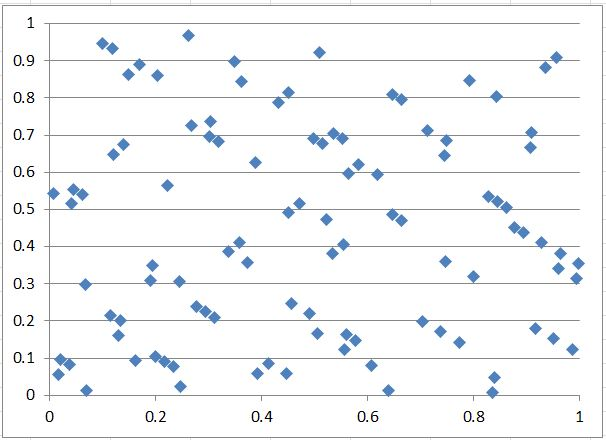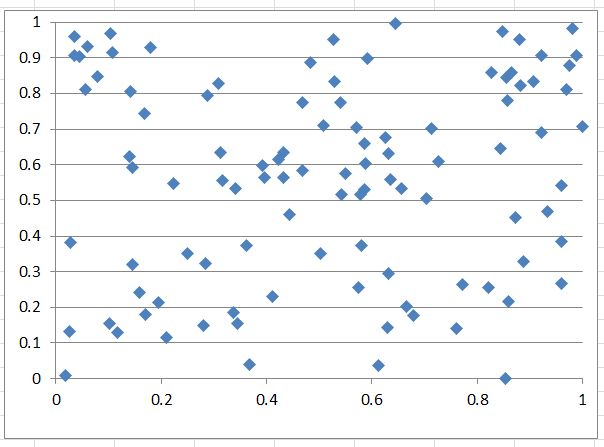100 point Excel scatter plot of dimensions 1x2:(left) quasi random; (right) pseudo random

The following charts compare a set of 500 two-dimensional quasi random vectors generated with {=rrand(1,2)} (left chart) with a set of 500 pairs of pseudo random numbers generated with =rand() (right chart):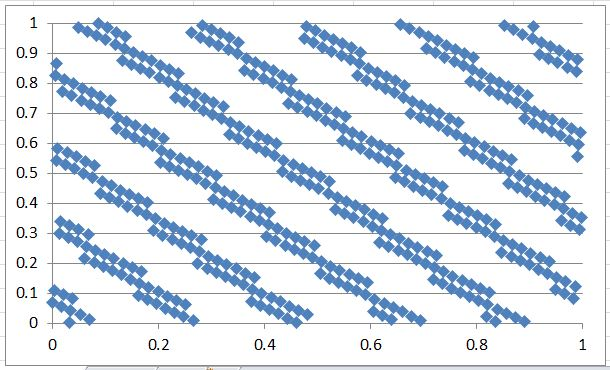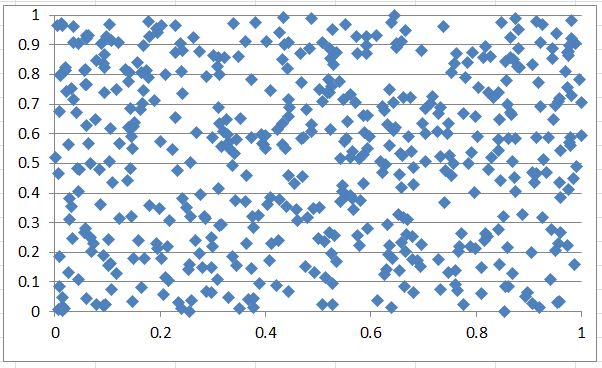500 point Excel scatter plot of two dimensions 1x2:(left) quasi random; (right) pseudo random

Note that gaps or holes are distributed more uniformly across space with the two-dimensional quasi random vectors than with pairs of pseudo random numbers. This is one reason why many theoretical and empirical results indicate that simulations based on quasi random vectors converge an order of magnitude faster than simulations based on sets of pseudo random numbers.

In multiple dimensional space, two-dimensional scatter plots (projections) show how quasi random numbers fill in space with different patterns.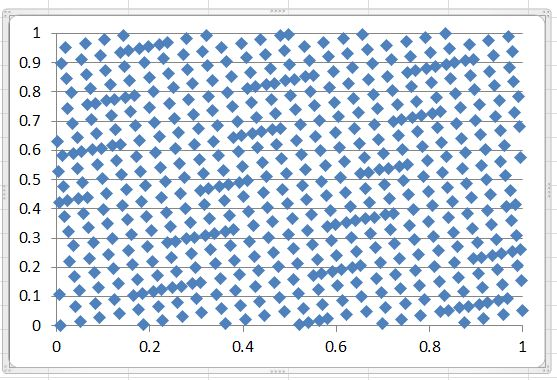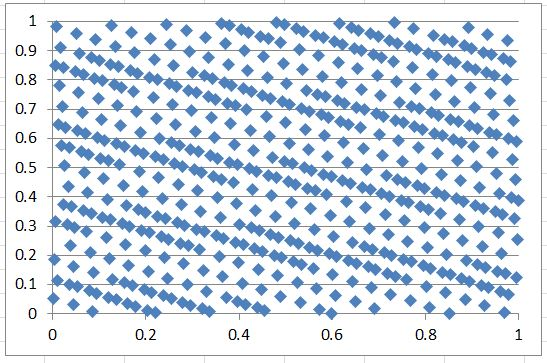Projections in two dimensions. 500 point Excel scatter plot of dimensions (left to right): 1x3; 1x4; 1x27.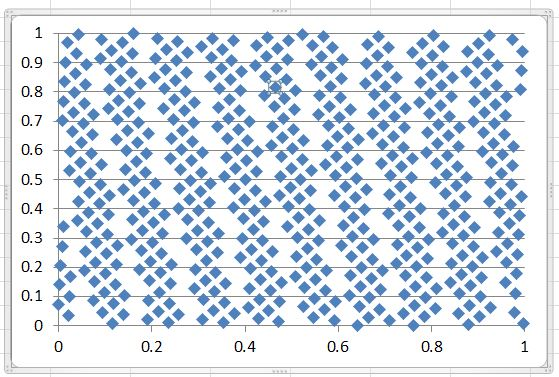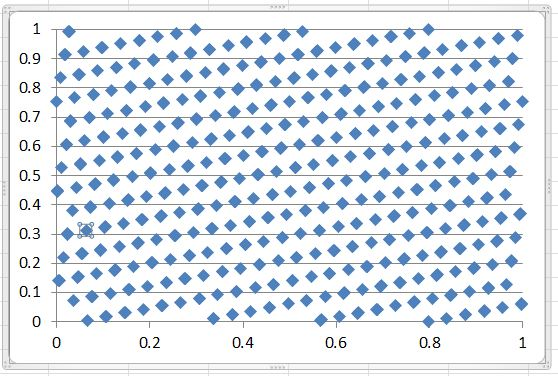Projections in two dimensions. 500 point Excel scatter plot of dimensions (left to right): 2x3; 5x27; 8x23.

RunRandom Instructions To generate a uniformly distributed random vector that updates with spreadsheet calculation:

1. Select a region of size myrows x mycols.
2. Insert the RRAND function as an array formula (Control-Shift-Enter).

The array function =RRAND(myrows, mycols) returns a uniformly distributed (between 0 and 1) quasi-random vector of dimension myrows * mycols.

RRAND is volatile - like Excel's rand() function: RRAND computes a new random vector at every spreadsheet recalculation.

To generate a uniformly distributed random vector that does not update with spreadsheet calculation:
1. Select a region of size myrows x mycols.
2. Insert the RRANDN function as an array formula (Control-Shift-Enter).

The array function =RRANDN(myrows, mycols) returns uniformly distributed (between 0 and 1) quasi-random vector of dimension myrows * mycols.

RRANDN is the "non-volatile" version of RRAND: RRANDN does not automatically recalculate.

To generate a zero-mean unit-variance normally distributed random vector that does updates with spreadsheet calculation:
1. Select a region of size myrows x mycols.
2. Insert the RRNORM function as an array formula (Control-Shift-Enter).

The array function RRNORM(myrows,mycols) returns standard (0 mean unit variance) normally distributed quasi-random vector of dimension myrows * mycols. RRNORM is volatile - like Excel's rand() function:

RRNORM computes a new random vector at every spreadsheet recalculation.

To generate a zero-mean unit-variance normally distributed random vector that does not update with spreadsheet calculation:
1. Select a region of size myrows x mycols.
2. Insert the RRNORMN function as an array formula (Control-Shift-Enter).

The array function RRNORMN(myrows,mycols) returns standard (0 mean unit variance) normally distributed quasi-random vector of dimension myrows * mycols.

RRNORMN is the "non-volatile" version of RRNORM: RRNORMN does not automatically recalculate.

The following charts compare a set of 500 two dimensional normally distributed (zero mean unit variance) quasi random vectors generated with {=rrnorm(1,2)} (left chart) with a set of 500 pairs of normally distributed (zero mean unit variance) pseudo random numbers generated with =NORMSINV(RAND()) (right chart):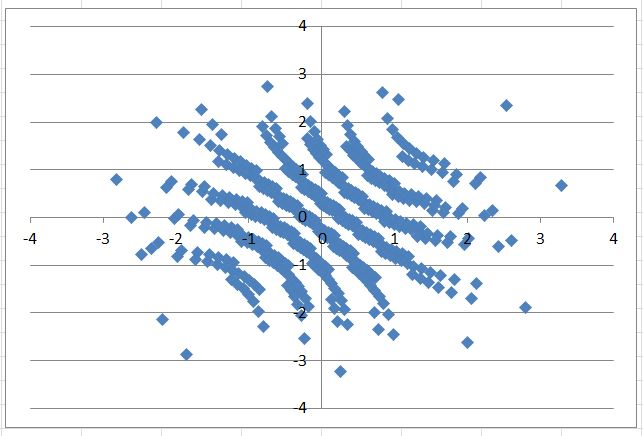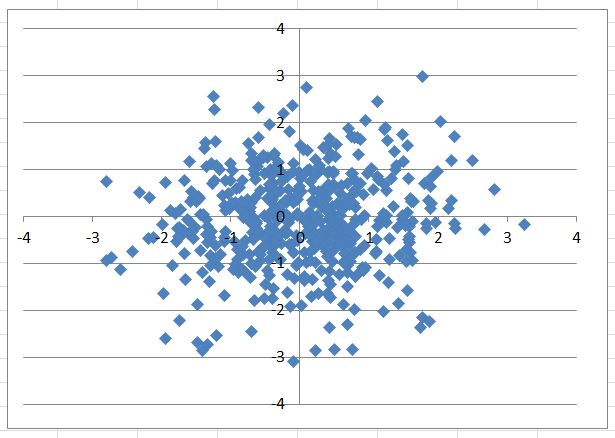500 point Scatter plot of dimensions 1x2:(left) standard normal quasi random; (right) standard normal pseudo random

To use the RunRandom functions from your own VBA Module:

1. Open your project module with the Excel VBA tools.
2. Navigate on the VBA Module Ribbon to: Tools=>References...
3. Search for "RunRandom" or "RunRandomStudent" on the list of "Available References."
4. Check the Box next to "RunRandom" or "RunRandomStudent".
5. Click OK. You can now call the RunRandom functions from your own VBA macro.
NOTE: For 64 bit versions of Excel, the total number of quasi-Random multidimensional vectors (independent of dimension) before recycling is approximately 2^63-1 ~ 9 x 10^18.